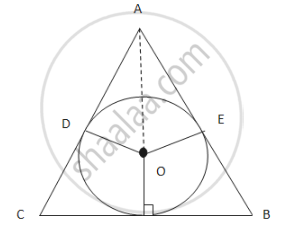# Prove that the Least Perimeter of an Isosceles Triangle in Which a Circle of Radius R Can Be Inscribed is - Mathematics and Statistics

Prove that the least perimeter of an isosceles triangle in which a circle of radius r can be inscribed is 6sqrt3 r.

#### SolutionLet ABC is an isosceles triangle with AB=AC=x and a circle with centre O and radius r is inscribed in the triangle. O,A and O,E and O,D are joined.From ΔABF,

AF^2+BF^2=AB^2

⇒(3r)^2+(y2)^2=x^2      .....(1)

Again,From ΔADO,(2r)^2=r^2+AD^2

⇒3r^2=AD^2

⇒AD=sqrt3r

Now, BD=BF and EC=FC (Since tangents drawn from an external point are equalNow, AD+DB=x

⇒(sqrt3r)+(y^2)=x

⇒y^2=x−sqrt3     .....(2)

∴(3r)^2+(x−sqrt3r)^2=x^2

⇒9r^2+x^2−2sqrt3rx+3r^2=x^2

⇒12r^2=2sqrt3rx

⇒6r=sqrt3x

⇒x=6r/sqrt3

Now, From (2),

y/2=6/sqrt3r−sqrt3r

⇒y/2=6/sqrt3r−sqrt3r

⇒y/2=((6sqrt3−3sqrt3)r)/3

⇒y/2=(3sqrt3r)/3

⇒y=2sqrt3r

Perimeter=2x+y

=2(6/sqrt3r)+2sqrt3r

=12/sqrt3r+2sqrt3r

=(12r+6r)/sqrt3

=18/sqrt3r

=(18xxsqrt3)/(sqrt3xxsqrt3)r

=6sqrt3r

Concept: Tangents and Normals
Is there an error in this question or solution?